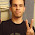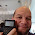## Thursday, May 10, 2012

### Calculus Revisited #18: Series

Welcome to Part 18 of our wonderful 21 part of our Calculus Revisited Series. I hope you are enjoying this series. An announcement: Part 21 will be a "catch all" section - covering some of the topics we don't get to in detail in this series.

The next three blog entries will be about series. Today, the basics.

Series:

A series is a sum of terms in a sequence. The series can be finite or infinite. An infinite series is convergent (has a value) if the following is true:

∑ a_n = S
n=i

and S < ∞

Here are some famous series:

Arithmetic Series:

a + (a + d) + (a + 2d) + (a + 3d) + ...

Sum for a finite arithmetic series:

n-1
∑ a + k * d
k=0

= a * n + (d * n * (n -1))/2

Geometric Series:

a + a * r + a * r^2 + a * r^3 + ...

Sum for an infinite geometric series, provided that |r| < 1:

∑ a * r^k = a / (1 - r)
k = 0

Harmonic Series:

∑ 1/n
n=1

= 1 + 1/2 + 1/3 + 1/4 + 1/5 + ....

There is no closed formula for a finite harmonic series. In addition the infinite harmonic series diverges (has no sum).

Series Properties:

∑ c * a(n) = c * ∑ a(n) (c is a constant)

∑ a(n) + b(n) = ∑ a(n) + ∑ b(n)

n
∑ k = n * (n + 1)/2
k=1

n
∑ k^2 = n * (n + 1) * (2n + 1)/6
k = 1

n
∑ k^3 = n^2 *(n + 1)^2 / 4
k = 1

Subtracting from a whole:
If t > 1:

k
∑ a(n) =
n = t

∑( a(n) for n = 1 to k) - ∑( a(n) for n = 1 to t - 1)

Problems

1. Calculate

6
∑ 50 - 2k
k = 0

This is an arithmetic series with n = 6 + 1 = 7, a = 50, and d = -2.

Then the sum is:
(50)(7) + (-2 * 7 * 6)/2 = 308

2. Show why the (infinite) harmonic series is divergent.

Here is one way:

Let S be the sum of the harmonic series, that is:

S = 1 + 1/2 + 1/3 + 1/4 + 1/5 + 1/6 + 1/7 + 1/8 + ...

Note that:
1 + 1/2 > 2/2
1/3 + 1/4 > 2/4
1/5 + 1/6 > 2/6
1/7 + 1/8 > 2/8
and so on...

Then we have 2/2 + 2/4 + 2/6 + 2/8 + ....

Which simplifies to 1 + 1/2 + 1/3 + 1/4 + .... = S

Which implies S > S, which is impossible.

The harmonic series is divergent.

3. Find the sum:

∑ .5^n + .3^n
n=0

We can first break the sum up:

∑(.5^n for n=0 to ∞) + ∑(.3^n for n=0 to ∞)

Both terms are geometric series. r = .5 for the first term and r = .3 for the second term.

Then:

∑(.5^n for n=0 to ∞) + ∑(.3^n for n=0 to ∞)
= 1 / (1 - .5) + 1 / (1 - .3)
= 1 / .5 - 1 / .7

4. Find the sum:

∑ 2 / (5^n)
n = 1

This looks like a geometric series with r = 1/5. If we can put the sum into it's proper form, perhaps by adding and subtracting 2 / 5^0:

∑( 2 / (5^n) for n = 1 to ∞)
= ∑ ( 2 / (5^n) for n = 0 to ∞) - 2 / 5^0
= ∑ ( 2 / (5^n) for n = 0 to ∞) - 2
= 2 / ( 1 - 1/5) - 2
= 2 / (4/5) - 2
= 5/2 - 2 = 1/2

5. Find the sum:

20
∑ k^2
k=10

Again, looks like a k^2 series, but use the subtraction from the whole technique and:

∑( k^2 for k = 10 to 20)
= ∑(k^2 for k = 1 to 20) - ∑(k^2 for k = 1 to 10 - 1)
= (20)(20 + 1)(2 * 20 + 1)/6 - (9)(9 + 1)(2 * 9 + 1)/6
= 17220 / 6 + 1710 / 6
= 15510 / 6 = 2585

Thank you once again for joining us. Next time we will work some series convergence tests.

Eddie

This blog is property of Edward Shore. © 2012

1.Interesting proof of the divergence of the harmonic series. I don't think I've seen it before.

The harmonic series diverges pretty slowly. You can think of this by finding the area under the curve of f(x) = 1/x. The anti-derivative is log(x), the natural logarithm.

The area under f(x) = 1/x from 1 to N will over estimate the sum 1 + 1/2 + 1/3 + ... + 1/N.

Sum | N
--------------------
5 | 148
10 | 22,026
15 | 3,269,017
20 | 485,165,195
25 | 7.200 * 10^10
50 | 5.185 * 10^21
100 | 2.688 * 10^43
150 | 1.394 * 10^65
200 | 7.226 * 10^86
225 | 5.203 * 10^97
--------------------

So it will actually take fewer terms to get to the sums in the table. For a sum of 5 you need to go out to about N = 83, for a sum of 10 you go out to N = 12,500 and for a sum of 15 you go out to 1.85 million.

The bigger the sum you want the more terms you need! As you can see the sum grows very slowly the further you go out, but it does diverge.

1.### Retro Review and Comparison: TI-82 Advanced

Retro Review and Comparison: TI-82 Advanced I can officially say that I have a French calculator.   Quick Facts: Models:  TI-82 Advanced Co...